https://github.com/wenet-e2e/wenet

• 端到端语音识别基础
• pytorch的实现介绍
• 进阶内容:cache

# 第1节: 端到端语音识别基础

1.对于每个句子扩展为单音素序列，用前向后向EM训练，得到单因素的hmm-单高斯model1。
2.用model1对句子做对齐，单高斯进行2倍分裂，更新模型，迭代这个对齐/分裂的过程n次，得到单因素的hmm-gmm模型model2.
3.用model2对句子做对齐，将音素根据上下文扩展为三音素，使用单高斯学习每个音素的决策树，，最后每个叶子结点对应一个单高斯. 得到一个三音素-hmm-单高斯模型model3
4.类似于第2步，用model3不停迭代分裂高斯，得到三音素hmm-gmm的model4
5. model4对句子做对齐，对齐数据用于帧级别NN训练.

• 直接以目标单元作为建模对象，比如中文使用字，英文使用字符或者BPE.
• 通过特殊的模型（目标函数），处理输入输出对齐未知的问题。

## CTC目标函数

CTC本质上对所有合法的输出和输入对齐方式进行了穷举，所谓合法，即对齐后的输出序列能够按CTC规则规约得到的原标注序列，则为合法对齐。

• 连续的相同字符进行合并
• 移除blank字符

...

CTC目标函数的思想是: 既然不知道哪种对齐关系是正确的，那就最大化所有合法CTC对齐的概率之和。所以对于这个样本，目标就是最大化如下概率。

P(出门问问|X) = P(出-门问问-问|X) + P(出出门问问-问|X)
+ P(出出出门问-问|X)+ ... + P(出--门问-问|X)

## Attention-based Encoder Decoder

Attention-based Encoder Decoder简称AED，也叫Seq2Seq框架，在ASR领域里，该框架也叫做LAS（Listen, Attend and Spell）。

AED的解码

CTC没有显示构建文本之间的关系，RNN-t模型是一种显示建模了文本关系的帧同步解码的模型。

## 神经网络类型

Transformer由多个Transformer Block堆叠，每个Block中会使用self-attention，res，relu，ff层。

Conformer由多个Conformer Block堆叠，每个Block中会使用conv，self-attention，res，relu，ff层。

# 第2节: Wenet中的神经网络设计与实现

## 模型入口 ASRModel

wenet/transformer/asr_model.py

### 模型定义

class ASRModel(torch.nn.Module)
def __init__():
self.encoder = encoder
self.decoder = decoder
self.ctc = ctc
self.criterion_att = LabelSmoothingLoss(...）# AED的loss


ASRModel的init中定义了encoder, decoder, ctc, criterion_att几个基本模块。其整体网络拓扑如下图所示。

• encoder是Shared Encoder，其中也包括了Subsample网络。
• decoder是Attention-based Decoder网络
• ctc是ctc Decoder网络（该decoder结构很简单，仅仅是前向网络和softmax）和ctc loss
• criterion_att是Attention-based Decoder对应的自回归似然loss，实际是一个LabelSmoothing的loss。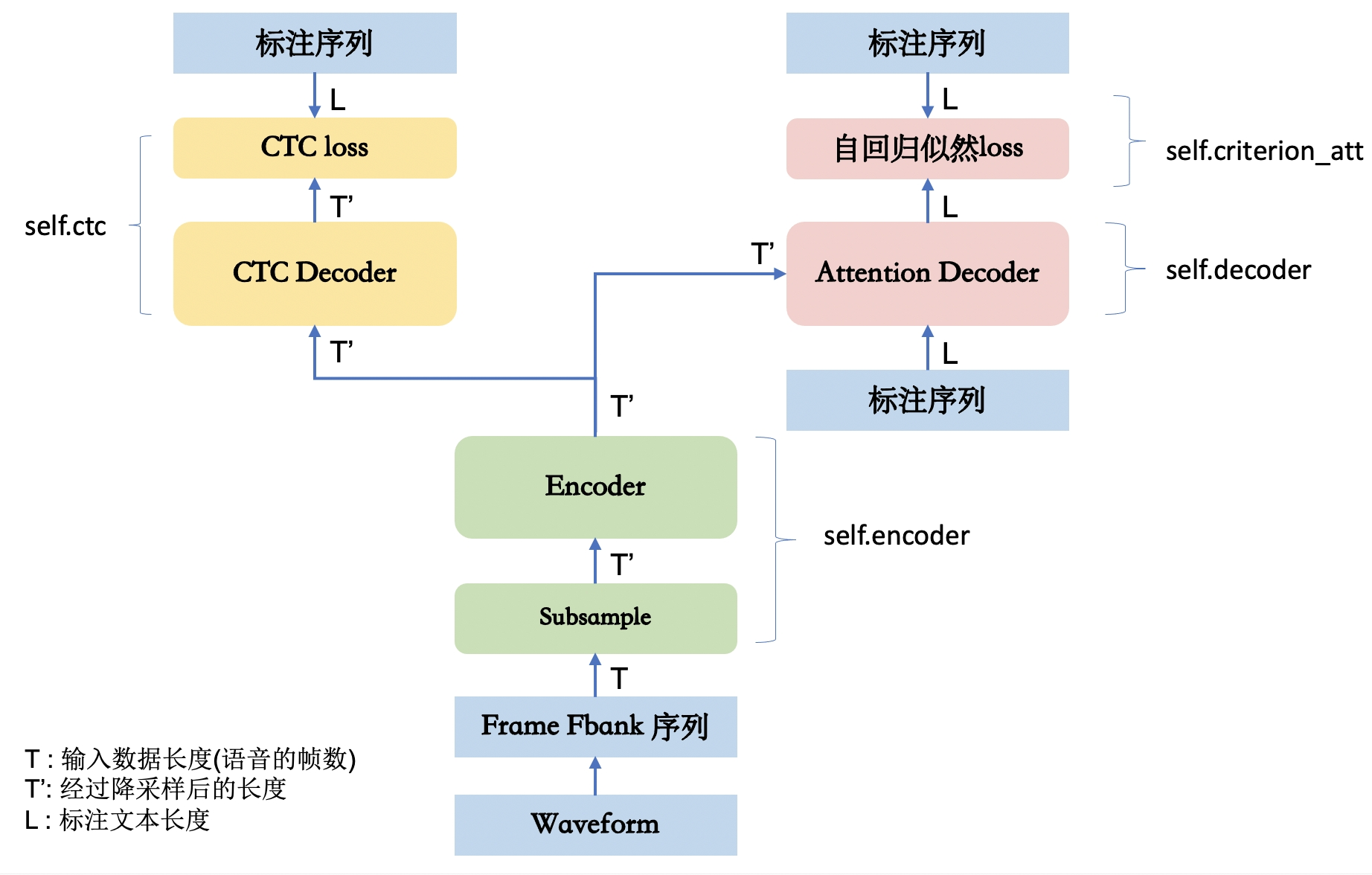ASRModel中的模块又有自己的子模块，可以通过print打印出完整的模型结构。

model = ASRModel(...)
print(model)


### 创建模型

def init_asr_model(config):


### 前向计算

pytorch框架下，只需定义模型的前向计算forword,。对于每个Module，可以通过阅读forward代码来学习其具体实现。

class ASRModel(torch.nn.Module):
def forward()
...
# Encoder

# Attention-decoder
text, text_lengths)
# CTC
loss_ctc = self.ctc(encoder_out, encoder_out_lens, text,text_lengths)
loss = self.ctc_weight * loss_ctc + (1 -self.ctc_weight) * loss_att
...


### 其他接口

ASRModel除了定义模型结构和实现前向计算用于训练外，还有两个功能：

• 提供多种python的解码接口
• 提供runtime中需要使用的接口

python解码接口

recognize() # attention decoder
attention_rescoring() # CTC + attention rescoring
ctc_prefix_beam_search() # CTC prefix beamsearch
ctc_greedy_search() # CTC greedy search


subsampling_rate()
right_context()
sos_symbol()
eos_symbol()
forward_encoder_chunk()
forward_attention_decoder()
ctc_activation()


• forward_attention_decoder()Attention Decoder的序列forward计算，非自回归模式
• ctc_activation()CTC Decoder forward计算
• forward_encoder_chunk()基于chunk的Encoder forward计算

## Encoder网络

wenet/transformer/encoder.py

Wenet的encoder支持Transformer和Conformer两种网络结构，实现时使用了模版方法的设计模式进代码复用。BaseEncoder中定义了如下统一的前向过程，由TransformerEncoder，ConformerEncoder继承BaseEncoder后分别定义各自的self.encoders的结构。

class BaseEncoder(torch.nn.Module):
def forward(...):
for layer in self.encoders:
if self.normalize_before:
xs = self.after_norm(xs)


• self.embed是Subsampling网络
• self.encoders是一组相同结构网络（Encoder Blocks）的堆叠

• forward_chunk_by_chunk，python解码时，模拟流式解码模式基于chunk的前向计算。
• forward_chunk, 单次基于chunk的前向计算，通过ASRModel导出为forward_encoder_chunk()供runtime解码使用。

### Subsampling网络

wenet/transformer/subsampling.py

• 2D-Conv: 输入数据看作是深度(通道数）为1，高度为F（Fbank特征维度，idim），宽度为T（帧数）的一张图.
• 1D-Conv: 输入数据看作是深度(通道数）为F（Fbank特征维度)，高度为1，宽度为T（帧数）的一张图.

Kaldi中著名的TDNN就是是1D-Conv，在Wenet中采用2D-Conv来实现降采样。

Wenet中提供了多个降采样的网络，这里选择把帧率降低4倍的网络Conv2dSubsampling4来说明。

class Conv2dSubsampling4(BaseSubsampling):
def __init__(self, idim: int, odim: int, dropout_rate: float,
pos_enc_class: torch.nn.Module):
"""Construct an Conv2dSubsampling4 object."""
super().__init__()
self.conv = torch.nn.Sequential(
torch.nn.Conv2d(1, odim, 3, 2),
torch.nn.ReLU(),
torch.nn.Conv2d(odim, odim, 3, 2),
torch.nn.ReLU(),
)
self.out = torch.nn.Linear(odim * (((idim - 1) // 2 - 1) // 2), odim)
self.pos_enc = pos_enc_class
# The right context for every conv layer is computed by:
# (kernel_size - 1) / 2 * stride  * frame_rate_of_this_layer
self.subsampling_rate = 4
# 6 = (3 - 1) / 2 * 2 * 1 + (3 - 1) / 2 * 2 * 2
self.right_context = 6


torch.nn.Conv2d(1, odim, kernel_size=3, stride=2)
torch.nn.Conv2d(odim, odim, kernel_size=3, stride=2)


def forward(...):
x = x.unsqueeze(1)  # (b, c=1, t, f)
x = self.conv(x)
b, c, t, f = x.size()
x = self.out(x.transpose(1, 2).contiguous().view(b, t, c * f))
x, pos_emb = self.pos_enc(x, offset)
return x, pos_emb, x_mask[:, :, :-2:2][:, :, :-2:2]

• x = x.unsqueeze(1) # (b, c=1, t, f) 增加channel维，以符合2dConv需要的数据格式。
• view(b, t, c * f) 将深度和高度合并平铺到同一维，然后通过self.out(）对每帧做Affine变换
• pos_enc(x, offset) 经过subsampling之后，帧数变少了，此时再计算Positional Eembedding。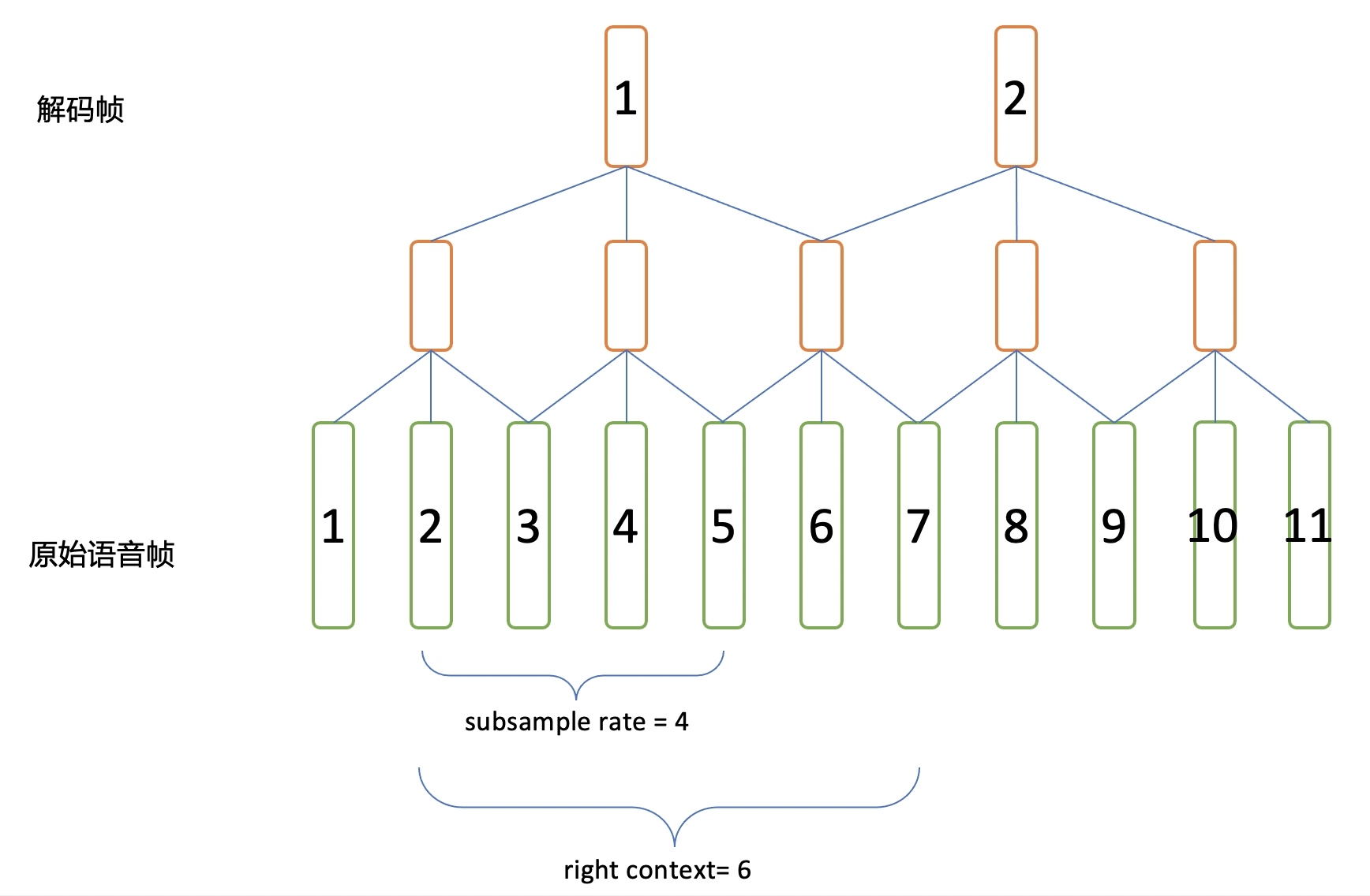self.subsampling_rate = 4
self.right_context = 6


• 第1个解码帧，需要依赖第1到第7个原始语音帧。
• 第2个解码帧，需要依赖第5到第11个原始语音帧。

subsampling_rate: 对于相邻两个解码帧，在原始帧上的间隔。

right_context: 对于某个解码帧，其对应的第一个原始帧的右侧还需要额外依赖多少帧，才能获得这个解码帧的全部信息。

# runtime/core/decoder/torch_asr_decoder.cc
if (!start_) {                      // First chunk
int context = right_context + 1;  // Add current frame
num_requried_frames = (opts_.chunk_size - 1) * subsampling_rate + context;
} else {
num_requried_frames = opts_.chunk_size * subsampling_rate;
}


### Encoder Block

wenet/transformer/encoder_layer.py

Transformer的self.encoders由一组TransformerEncoderLayer组成

self.encoders = torch.nn.ModuleList([
TransformerEncoderLayer(
output_size,
attention_dropout_rate),
PositionwiseFeedForward(output_size, linear_units,
dropout_rate), dropout_rate,
normalize_before, concat_after) for _ in range(num_blocks)
])


Conformer的self.encoders由一组ConformerEncoderLayer组成

self.encoders = torch.nn.ModuleList([
ConformerEncoderLayer(
output_size,
PositionwiseFeedForward(*positionwise_layer_args),
PositionwiseFeedForward(*positionwise_layer_args)
if macaron_style else None,
ConvolutionModule(*convolution_layer_args)
if use_cnn_module else None,
dropout_rate,
normalize_before,
concat_after,
) for _ in range(num_blocks)
])


• PositionwiseFeedForward
• ConvolutionModule

conformer论文中conformer block的结构如图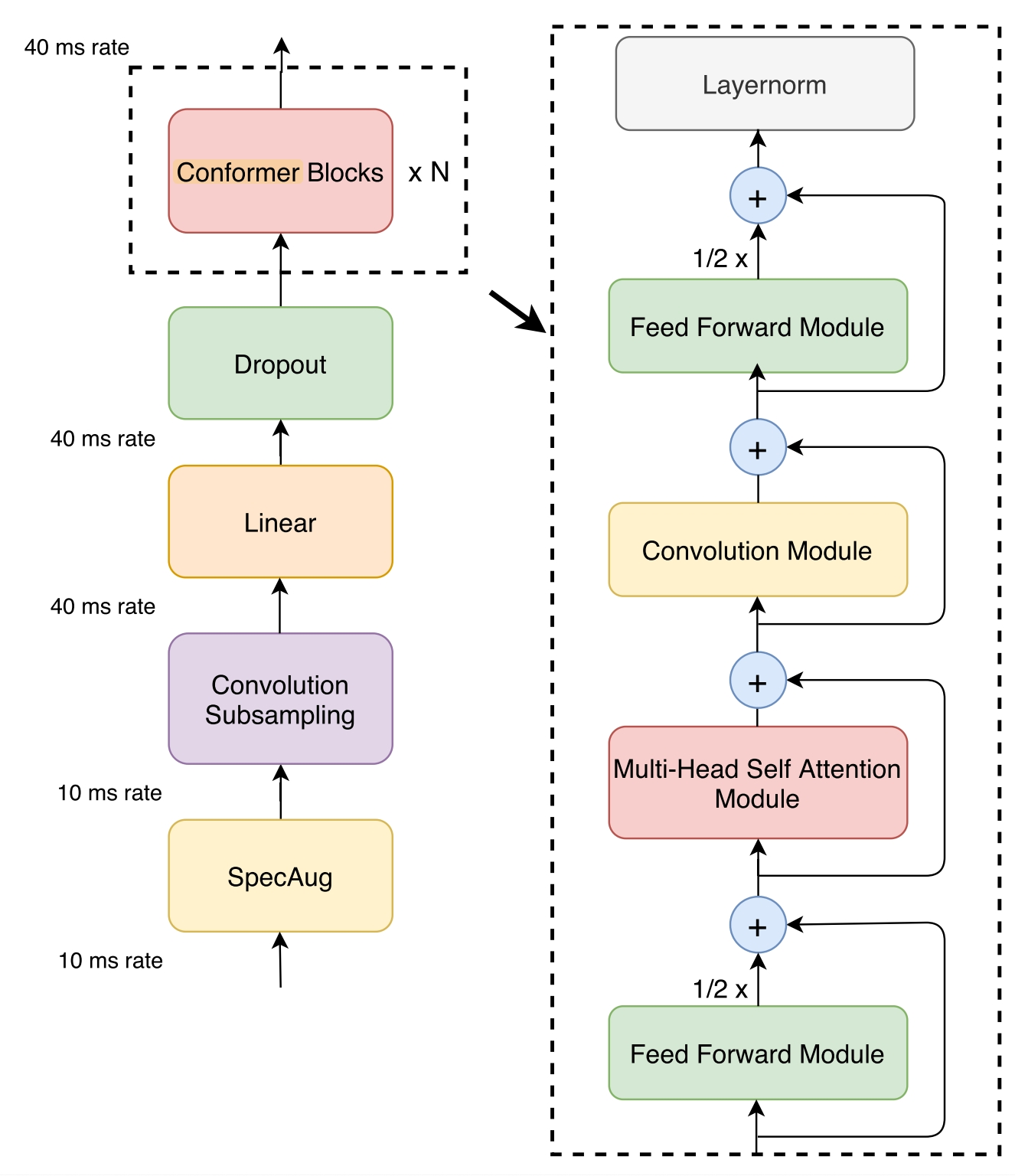class ConformerEncoderLayer(nn.Module):
def forward(...):
residual = x
x = self.norm_ff_macaron(x)
x = self.feed_forward_macaron(x)
x = residual + 0.5 * self.dropout(x)

residual = x
x = self.norm_mha(x)
x_att = self.self_attn(x, x, x, pos_emb, mask)
x = residual + self.dropout(x_att)

residual = x
x = self.norm_conv(x)
x = residual + self.dropout(x)

residual = x
x = self.norm_ff(x)
x = self.feed_forward(x)
x = residual + 0.5 * self.dropout(x)

x = self.norm_final(x)


wenet/transformer/attention.py
attention.py中提供了两种attention的实现，MultiHeadedAttentionRelPositionMultiHeadedAttention

MultiHeadedAttention用于Transformer。RelPositionMultiHeadedAttention用于Conformer。

Conformer Block - PositionwiseFeedForward
wenet/transformer/positionwise_feed_forward.py

PositionwiseFeedForward，对各个帧时刻输入均使用同一个矩阵权重去做前向Affine计算，即通过一个[H1, H2]的的前向矩阵，把[B, T, H1]变为[B，T，H2]。

Conformer Block - ConvolutionModule
wenet/transformer/convolution.py

ConvolutionModule结构如下

Wenet中使用了因果卷积(Causal Convolution)，即不看右侧上下文，这样无论模型含有多少卷积层，对右侧的上下文都无依赖。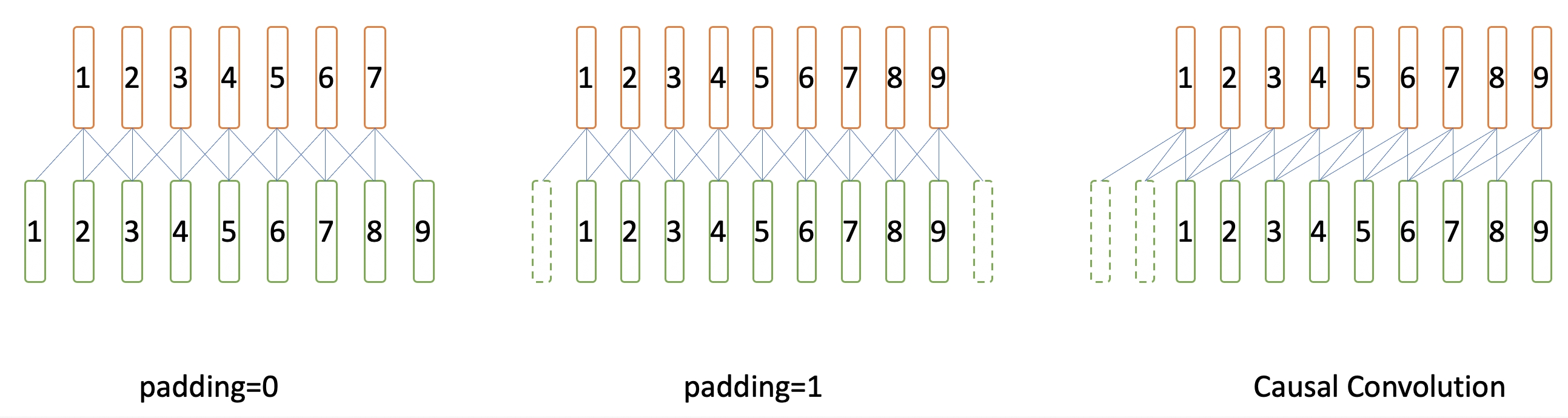if causal: # 使用因果卷积
self.lorder = kernel_size - 1 # 因果卷积左侧手动padding的长度
else: # 使用标准卷积
# kernel_size should be an odd number for none causal convolution
assert (kernel_size - 1) % 2 == 0
self.lorder = 0


if self.lorder > 0:
if cache is None:
x = nn.functional.pad(x, (self.lorder, 0), 'constant', 0.0)


## CTC Loss

wenet/transformer/ctc.py

CTC Loss包含了CTC decoder和CTC loss两部分，CTC decoder仅仅对Encoder做了一次前向线性计算，然后计算softmax.

    # hs_pad: (B, L, NProj) -> ys_hat: (B, L, Nvocab)
# ys_hat: (B, L, D) -> (L, B, D)
ys_hat = ys_hat.transpose(0, 1)
ys_hat = ys_hat.log_softmax(2)
loss = self.ctc_loss(ys_hat, ys_pad, hlens, ys_lens)
# Batch-size average
loss = loss / ys_hat.size(1)
return loss


CTC loss的部分则直接使用的torch提供的函数 torch.nn.CTCLoss.

self.ctc_loss = torch.nn.CTCLoss(reduction=reduction_type)


## Attention based Decoder Loss

wenet/transformer/label_smoothing_loss.py

Attention-based Decoder的Loss是在最大化自回归的概率，在每个位置计算模型输出概率和样本标注概率的Cross Entropy。这个过程采用teacher forcing的方式，而不采用scheduled sampling。

## 网络的完整结构

ASRModel(
(encoder): ConformerEncoder(
(global_cmvn): GlobalCMVN()
(embed): Conv2dSubsampling4(
(conv): Sequential(
(0): Conv2d(1, 256, kernel_size=(3, 3), stride=(2, 2))
(1): ReLU()
(2): Conv2d(256, 256, kernel_size=(3, 3), stride=(2, 2))
(3): ReLU()
)
(out): Sequential(
(0): Linear(in_features=4864, out_features=256, bias=True)
)
(pos_enc): RelPositionalEncoding(
(dropout): Dropout(p=0.1, inplace=False)
)
)
(after_norm): LayerNorm((256,), eps=1e-12, elementwise_affine=True)
(encoders): ModuleList(
(0): ConformerEncoderLayer(
(linear_q): Linear(in_features=256, out_features=256, bias=True)
(linear_k): Linear(in_features=256, out_features=256, bias=True)
(linear_v): Linear(in_features=256, out_features=256, bias=True)
(linear_out): Linear(in_features=256, out_features=256, bias=True)
(dropout): Dropout(p=0.0, inplace=False)
(linear_pos): Linear(in_features=256, out_features=256, bias=False)
)
(feed_forward): PositionwiseFeedForward(
(w_1): Linear(in_features=256, out_features=2048, bias=True)
(activation): Swish()
(dropout): Dropout(p=0.1, inplace=False)
(w_2): Linear(in_features=2048, out_features=256, bias=True)
)
(feed_forward_macaron): PositionwiseFeedForward(
(w_1): Linear(in_features=256, out_features=2048, bias=True)
(activation): Swish()
(dropout): Dropout(p=0.1, inplace=False)
(w_2): Linear(in_features=2048, out_features=256, bias=True)
)
(conv_module): ConvolutionModule(
(pointwise_conv1): Conv1d(256, 512, kernel_size=(1,), stride=(1,))
(depthwise_conv): Conv1d(256, 256, kernel_size=(15,), stride=(1,), groups=256)
(norm): LayerNorm((256,), eps=1e-05, elementwise_affine=True)
(pointwise_conv2): Conv1d(256, 256, kernel_size=(1,), stride=(1,))
(activation): Swish()
)
(norm_ff): LayerNorm((256,), eps=1e-12, elementwise_affine=True)
(norm_mha): LayerNorm((256,), eps=1e-12, elementwise_affine=True)
(norm_ff_macaron): LayerNorm((256,), eps=1e-12, elementwise_affine=True)
(norm_conv): LayerNorm((256,), eps=1e-12, elementwise_affine=True)
(norm_final): LayerNorm((256,), eps=1e-12, elementwise_affine=True)
(dropout): Dropout(p=0.1, inplace=False)
(concat_linear): Linear(in_features=512, out_features=256, bias=True)
)
...
)
)
(decoder): TransformerDecoder(
(embed): Sequential(
(0): Embedding(4233, 256)
(1): PositionalEncoding(
(dropout): Dropout(p=0.1, inplace=False)
)
)
(after_norm): LayerNorm((256,), eps=1e-12, elementwise_affine=True)
(output_layer): Linear(in_features=256, out_features=4233, bias=True)
(decoders): ModuleList(
(0): DecoderLayer(
(linear_q): Linear(in_features=256, out_features=256, bias=True)
(linear_k): Linear(in_features=256, out_features=256, bias=True)
(linear_v): Linear(in_features=256, out_features=256, bias=True)
(linear_out): Linear(in_features=256, out_features=256, bias=True)
(dropout): Dropout(p=0.0, inplace=False)
)
(linear_q): Linear(in_features=256, out_features=256, bias=True)
(linear_k): Linear(in_features=256, out_features=256, bias=True)
(linear_v): Linear(in_features=256, out_features=256, bias=True)
(linear_out): Linear(in_features=256, out_features=256, bias=True)
(dropout): Dropout(p=0.0, inplace=False)
)
(feed_forward): PositionwiseFeedForward(
(w_1): Linear(in_features=256, out_features=2048, bias=True)
(activation): ReLU()
(dropout): Dropout(p=0.1, inplace=False)
(w_2): Linear(in_features=2048, out_features=256, bias=True)
)
(norm1): LayerNorm((256,), eps=1e-12, elementwise_affine=True)
(norm2): LayerNorm((256,), eps=1e-12, elementwise_affine=True)
(norm3): LayerNorm((256,), eps=1e-12, elementwise_affine=True)
(dropout): Dropout(p=0.1, inplace=False)
(concat_linear1): Linear(in_features=512, out_features=256, bias=True)
(concat_linear2): Linear(in_features=512, out_features=256, bias=True)
)
...
)
)
(ctc): CTC(
(ctc_lo): Linear(in_features=256, out_features=4233, bias=True)
(ctc_loss): CTCLoss()
)
(criterion_att): LabelSmoothingLoss(
(criterion): KLDivLoss()
)
)


• decoder的自回归依赖关系如何表达
• chunk-based的encoder如何实现

Attention Loss

    ignore = target == self.padding_idx  # (B,)
total = len(target) - ignore.sum().item()
target = target.masked_fill(ignore, 0)  # avoid -1 index
true_dist.scatter_(1, target.unsqueeze(1), self.confidence)
kl = self.criterion(torch.log_softmax(x, dim=1), true_dist)
denom = total if self.normalize_length else batch_size


CTC loss

torch.nn.CTCLoss接口支持指定Batch内各个输出序列的长度hlens和各个标注序列的长度ys_lens

self.ctc_loss = torch.nn.CTCLoss(reduction=reduction_type)
loss = self.ctc_loss(ys_hat, ys_pad, hlens, ys_lens)


# wenet/transformer/asr_model.py


# wenet/utils/executor.py
key, feats, target, feats_lengths, target_lengths = batch


• Encoder的输入
• Decoder的输入

• 序列mask,（Batch, Length）， 每个 (Length,) 中值为1的位置代表了本序列要考虑的部分。
• Attention mask,（Batch, L1, L2），每个（L1，L2) 用于约束L1中的哪些位置只能对于L2中的哪些位置进行attention操作。

## 问题3: Chunk-Based Model

wenet/transformer/encoder.py
def forward()
...
self.use_dynamic_chunk,
self.use_dynamic_left_chunk,
decoding_chunk_size,
self.static_chunk_size,
num_decoding_left_chunks)
for layer in self.encoders:


1 1 1 1 1 1 1 0 0 0 0


1   1   1   1   0
1       1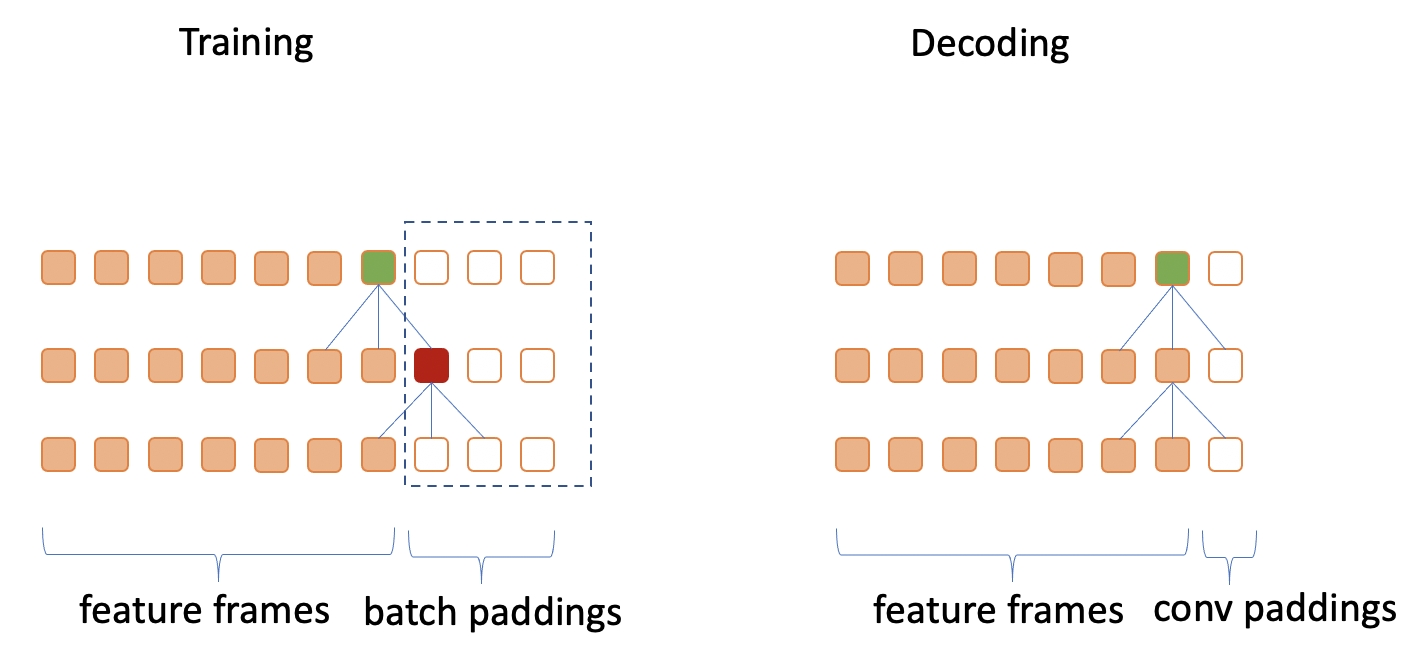# wenet/transformer/convolution.py
# def forward():


• Encoder中的self-attention
• Decoder中的self-attention
• Decoder中的cross-attention

# wenet/transformer/attention.py
def forward(self, query: torch.Tensor, key: torch.Tensor,
value: torch.Tensor,
"""Compute scaled dot product attention.

Args:
query (torch.Tensor): Query tensor (#batch, time1, size).
key (torch.Tensor): Key tensor (#batch, time2, size).
value (torch.Tensor): Value tensor (#batch, time2, size).
(#batch, time1, time2).
1.When applying cross attention between decoder and encoder,
the batch padding mask for input is in (#batch, 1, T) shape.
2.When applying self attention of encoder,
the mask is in (#batch, T, T)  shape.
3.When applying self attention of decoder,
the mask is in (#batch, L, L)  shape.
4.If the different position in decoder see different block
of the encoder, such as Mocha, the passed in mash could be
in (#batch, L, T) shape. But there is no such case in current
Wenet.
"""


# wenet/transformer/attention.py
def forward_attention ():
...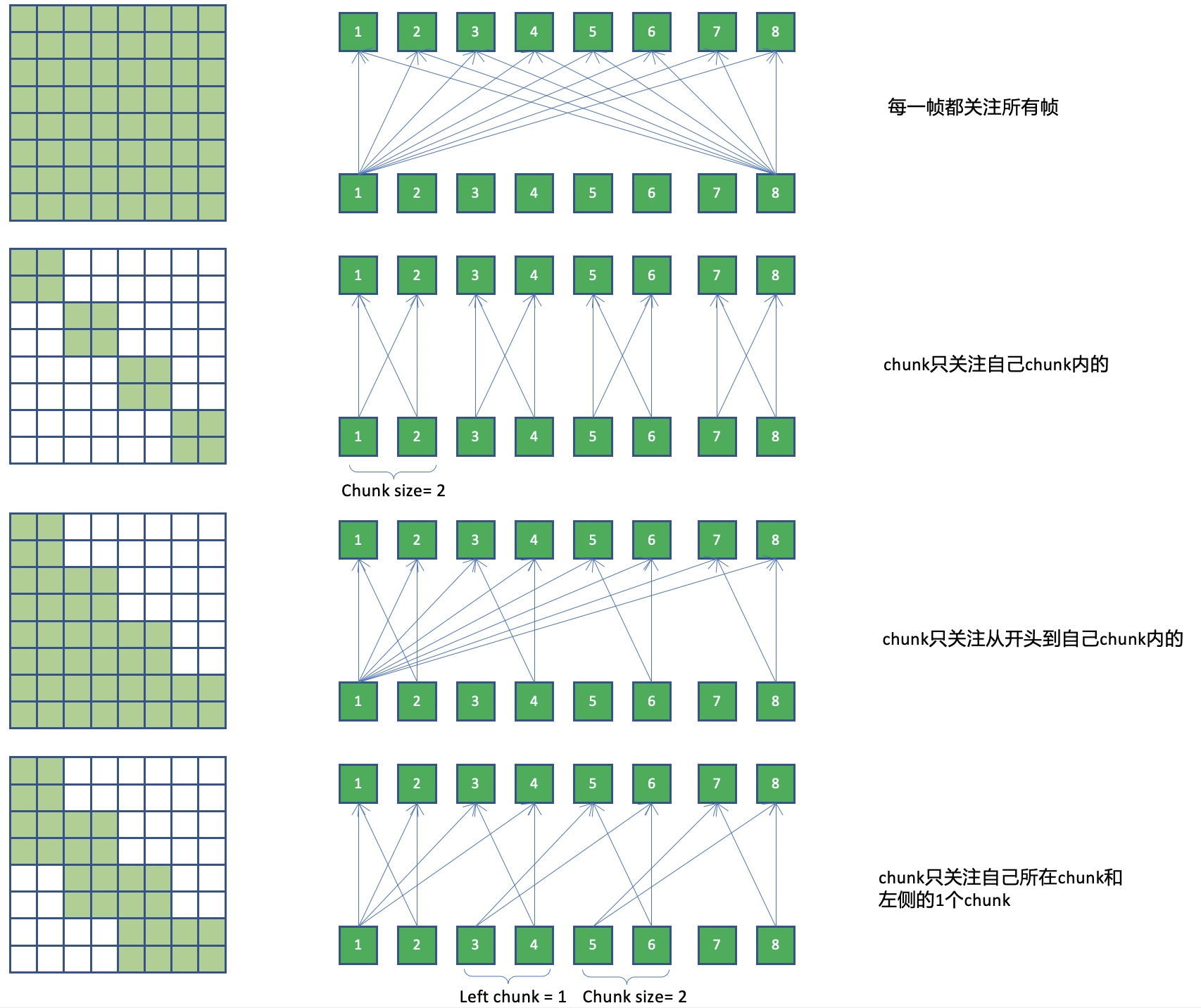def add_optional_chunk_mask(xs: torch.Tensor, masks: torch.Tensor,
use_dynamic_chunk: bool,
use_dynamic_left_chunk: bool,
decoding_chunk_size: int, static_chunk_size: int,
num_decoding_left_chunks: int):

• 如果 use_dynamic_left_chunk=True， 各的batch使用随机的的left chunk长度依赖
• 如果 use_dynamic_left_chunk=False， 各的batch使用均依赖开头到当前chunk
• use_dynamic_chunk = false, static_chunk_size <= 0. 使用full-attention.
• use_dynamic_chunk = false, static_chunk_size > 0. 使用固定的chunk mask.

Decoder涉及到两种Attention。 self.self_attn是decoder上的self attention。 self.src_attn是decoder和encoder的cross attention。

# wenet/transformer/decoder_layer.py
def forward():
...
...


Self attention

wenet/transformer/decoder.py
# m: (1, L, L)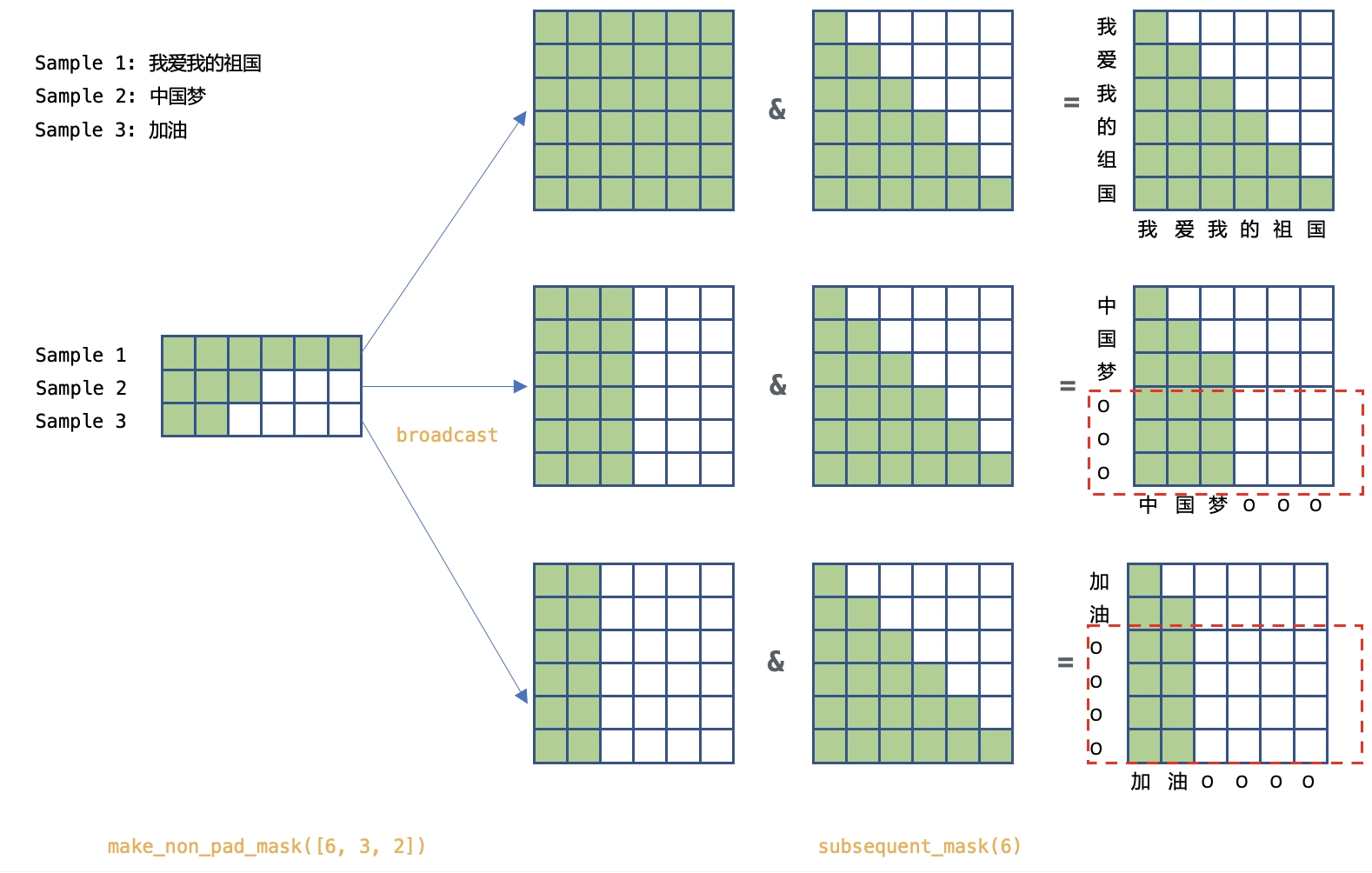Cross attention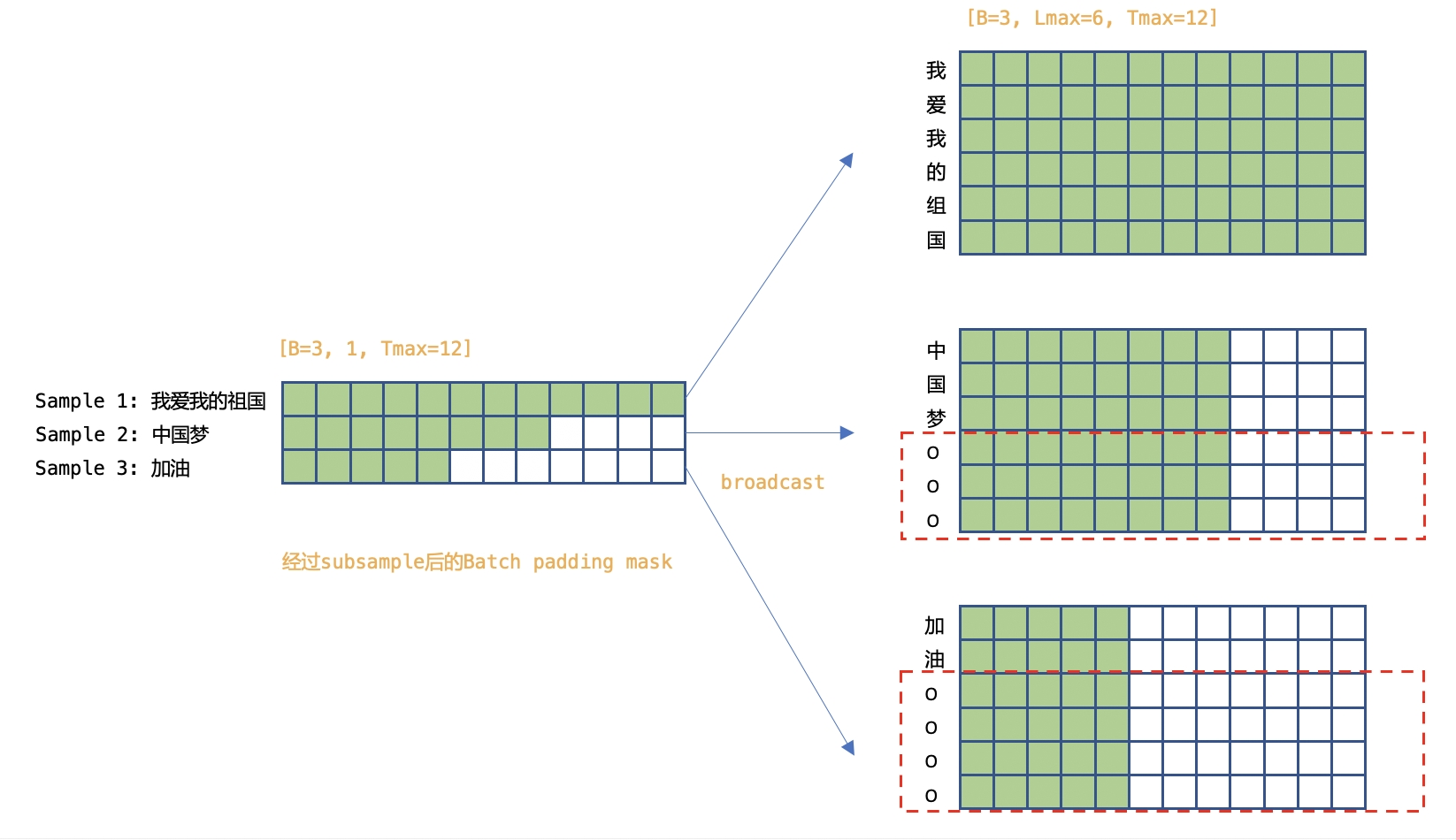Decoder中每一层中，均需要计算如上两个attention，从网络视角来看，如下图所示。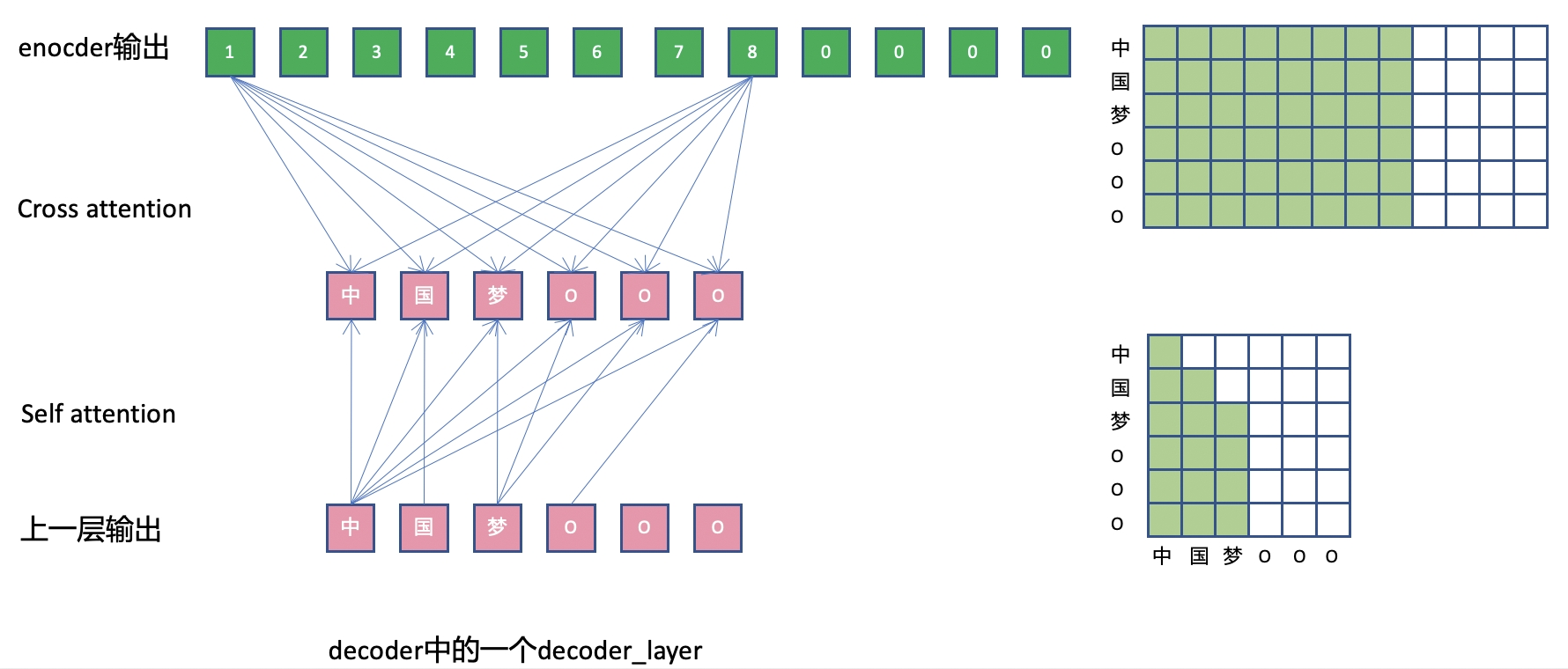# 第4节: 进阶话题:Cache

• 对于CTC decoder，由于是线性层，不需要cache。
• 对于AED decoder，是在计算完整个序列的encoder输出后进行rescoring，不涉及chunk。

## Runtime流式解码

asr_model.py中的forward_encoder_chunk()通过jit导出，用于C++ runtime，其内部使用了encoder.py中的forward_chunk()函数。

# wenet/transformer/asr_model.py

@torch.jit.export
def forward_encoder_chunk()


## Python流式解码

recognize() -> _forward_encoder() -> BaseEncoder.forward_chunk_by_chunk()


forward_chunk_by_chunk()的内部也是使用的forward_chunk()函数。

## BaseEncoder.forward_chunk()分析

forward_chunk()是对单个chunk进行前向计算的核心函数。下面从该函数的内容来了解cache的实现。

# wenet/transformer/encoder.py
def forward_chunk(
self,
xs: torch.Tensor,
offset: int,
required_cache_size: int,
subsampling_cache: Optional[torch.Tensor] = None,
elayers_output_cache: Optional[List[torch.Tensor]] = None,
conformer_cnn_cache: Optional[List[torch.Tensor]] = None,
) -> Tuple[torch.Tensor, torch.Tensor, List[torch.Tensor],
List[torch.Tensor]]:


xs是当前的chunk输入，由于对于单个chunk的前向计算，需要之前的chunk的计算得到的信息，因此这里需要传入相关的三个cache信息。

• subsampling_cache:torch.Tensor subsampling的输出的cache。即第一个conformer block的输入。
• elayers_output_cache:List[torch.Tensor] 第1个到最后1个conformer block的输出的cache。也就是第2个conformer block的输入和CTC层的输入
• conformer_cnn_cache:List[torch.Tensor] conformer block里的conv层的左侧依赖的输入cache

cache的大小

• subsampling_cache和elayers_output_cache的大小 由self-attention是对左侧的依赖长度required_cache_size决定。 decoding_chunk_size是解码帧级别的chunk大小, num_decoding_left_chunks是self-attention依赖的左侧chunk数。
required_cache_size = decoding_chunk_size * num_decoding_left_chunks

• conformer_cnn_cache的大小和required_cache_size无关，由casual网络的左侧上下文lorder决定。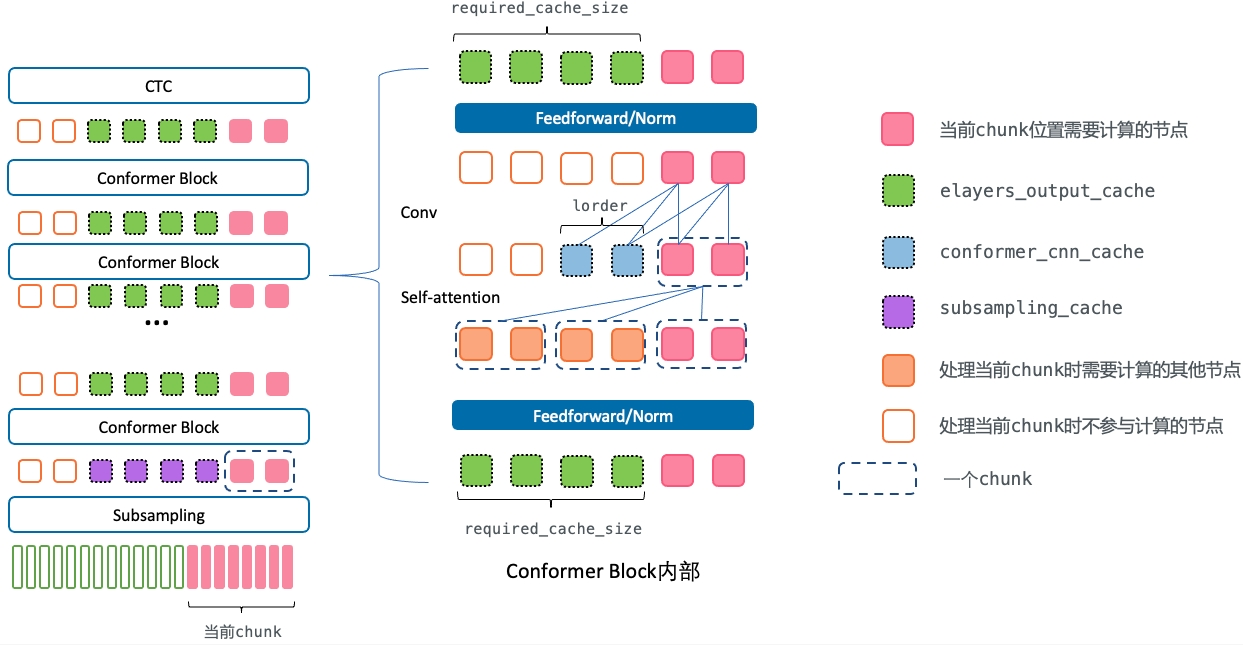### offset

xs, pos_emb, _ = self.embed(xs, tmp_masks, offset)


### subsampling内部

subsampling内部的计算虽然存在冗余，但是不进行cache。一个是其实现比较复杂，另一个原因是subsampling的计算量占比不大。

### subsampling_cache

subsampling的输出的cache。即第一个conformer block的输入。

if subsampling_cache is not None:
cache_size = subsampling_cache.size(1)
# xs是第一个conformer block的输入
xs = torch.cat((subsampling_cache, xs), dim=1)
else:
cache_size = 0
pos_emb = self.embed.position_encoding(offset - cache_size, xs.size(1))
if required_cache_size < 0:
next_cache_start = 0
elif required_cache_size == 0:
next_cache_start = xs.size(1)
else:
next_cache_start = max(xs.size(1) - required_cache_size, 0)
# 更新subsampling_cache
r_subsampling_cache = xs[:, next_cache_start:, :]


### elayers_output_cache

for i, layer in enumerate(self.encoders):
attn_cache = elayers_output_cache[i]
cnn_cache = conformer_cnn_cache[i]
xs, _, new_cnn_cache = layer(xs,
pos_emb,
output_cache=attn_cache,
cnn_cache=cnn_cache)
# 更新elayers_output_cache
r_elayers_output_cache.append(xs[:, next_cache_start:, :])


# wenet/transformer/encoder.py BaseEncoder.forward_chunk()
# 第一个conformer block输入的xs
xs = torch.cat((subsampling_cache, xs), dim=1)

# wenet/transformer/encoder_layer.py ConformerEncoderLayer.forward()
# 之后的conformer block输入的xs
if output_cache is not None:
x = torch.cat([output_cache, x], dim=1)


layer()对应着wenet/transformer/encoder_layer.py中的ConformerEncoderLayer.forward()。下面是其具体过程。

# 计算feedforwad/res/norm(包含当前chunk和左侧num_decoding_left_chunks个chunk)

# 使用cache时，只要计算当前chunk x_q的self-attentionattention和residual

chunk = x.size(1) - output_cache.size(1)
x_q = x[:, -chunk:, :]

# 只选择当前chunk对应的部分做residual计算
residual = residual[:, -chunk:, :]

# 使用当前chunk的x_q去和其依赖的x做attention
x = residual + self.dropout(self.self_attn(x_q, x, x, mask))

# 仅计算计算当前chunk的conv

x = self.norm2(x)
x = residual + self.dropout(self.feed_forward(x))

# chunk的输出和cache拼在一起，作为网络的最终输出。
x = torch.cat([output_cache, x], dim=1)


### conformer_cnn_cache

conformer block里的conv层的左侧依赖的输入cache。

conformer_cnn_cache大小为lorder，即因果卷积左侧依赖，。

# wenet/transformer/encoder_layer.py ConformerEncoderLayer.forward()
# conformer_cnn_cache通过ConvolutionModule.forward()返回的新cache来更新

# wenet/transformer/convolution.py ConvolutionModule.forward()